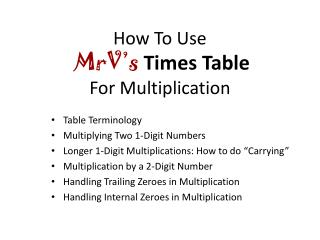Download PresentationHow To Use MrV’s Times Table For Multiplication

# How To Use MrV’s Times Table For Multiplication

Download Presentation## How To Use MrV’s Times Table For Multiplication

- - - - - - - - - - - - - - - - - - - - - - - - - - - E N D - - - - - - - - - - - - - - - - - - - - - - - - - - -
##### Presentation Transcript

1. How To UseMrV’sTimes TableFor Multiplication Table Terminology Multiplying Two 1-Digit Numbers Longer 1-Digit Multiplications: How to do “Carrying” Multiplication by a 2-Digit Number Handling Trailing Zeroes in Multiplication Handling Internal Zeroes in Multiplication

2. Table Terminology: Row, Cell, Factor, Product There are 9 table Rows,Row 1 through Row 9 Each Cellholds 3 numbers:The Product(big number) and its 2 Factors For example, this is Row 5: 6 5 In this Cell, the Product is 30 and theFactors are 5 and 6. Each Row has 10 Cells,Cell 0 thru Cell 9. For example, this is Cell 6 inRow 5.

3. Multiplying Two 1-digit Numbers 7 • 8=? 7 •8 1. Find the Rowfor the first Factor In this case, it’s Row 7: 3. The big number above them is their Product 7 times 8is 56 56 2. Look across the Row until you see both Factors on the bottom In this case, it’s 7 times 8

4. Ok, let’s try a few: Click when you find the Product 7 • 9= 7•9 9 • 4= 24 9•4 30 5 • 6= 5•6 63 3 • 8= 3•8 36

5. Longer 1-Digit Multiplications: How to do “Carrying” 4 • 582 = ? In this case, 4 is the multiplier. Leave some space on the top. We will use MrV’sRow 4: If necessary, rewrite the numbers in Vertical Format: Use the shorter number as multiplier. Neatness will improve accuracy. multiplicand ᵡ multiplier product 4 x 8 is 32 (move the 2 to the product and carry the 3 to over the 5) Starting from the right,4 x 2 is 8 (move the 8 to the product) 4 x 5 is 20, +3 is 23 (move the 23 to the product)

6. First, we’ll need Row 5: 5x7 then 5x7 then 5x5 then 5x9 Next, we’ll need Row 3: 3x7 then 3x7 then 3x5 then 3x9

7. Now, we’ll only need Row 7: 7x4 then 7x7 then 7x2